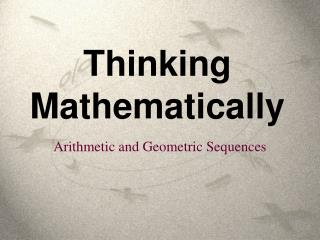DownloadDownload PresentationThinking Mathematically

# Thinking Mathematically

Download Presentation## Thinking Mathematically

- - - - - - - - - - - - - - - - - - - - - - - - - - - E N D - - - - - - - - - - - - - - - - - - - - - - - - - - -
##### Presentation Transcript

1. Thinking Mathematically Arithmetic and Geometric Sequences

2. 8 2 5 3 1 1 a3= a4= a6= a5= a1 a2 a3+ a4 a1+ a2 a4+ a5 a2+ a3 “Sequences” A number “sequence” is a list of numbers that are taken in the order in which they are written, normally from left to right. The following sequence is called the “Fibonacci” sequence. After the first two numbers, each number is the sum of the previous two. . . .

3. -5 -3 -1 5 3 1 a1 a6= a5= a4= a3= a2= a4+ 2 a5+ 2 a3+ 2 a2+ 2 a1+ 2 “Arithmetic” Sequences An “arithmetic” sequence is one in which each number is obtained from the previous one by adding a fixed amount. The fixed amount that is added to each term in an arithmetic sequence is called the “common difference” d. . . .

4. Example: 4, 7, 10, 13, 16, 19, . . . The 21th term will be a21 = a1+ (20 x d) = 4 + (20 x 3) = 4 + 60 = 64. “Arithmetic” Sequences The general term (the nthterm) of an arithmetic sequence can be found from the first term, a1, and the common difference, d. an = a1+ (n - 1) x d In this sequence a1 = 4 and d = 3.

5. 1 3 9 27 81 243 a1 a3= a4= a5= a6= a2= a2x3 a3x3 a1x3 a4x3 a5x3 “Geometric” Sequences An “geometric” sequence is one in which each number is obtained from the previous one by multiplying by a fixed amount. The fixed amount that each term is multiplied by to obtain the next term is called the “common ratio” r. . . .

6. Example: 3, 6, 12, 24, 48, 96, . . . The 8th term will be a8 = a1x r 7 = 3 x 27 = 3 x 128 = 384 “Geometric” Sequences The general term (the nthterm) of a geometric sequence can be found from the first term, a1, and the common difference, r. an = a1x r n-1 In this sequence a1 = 3 and r = 2.

7. Thinking Mathematically Arithmetic and Geometric Sequences